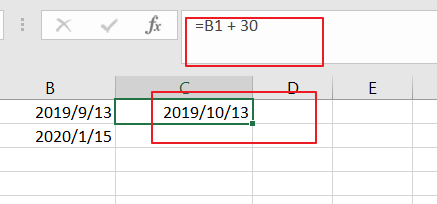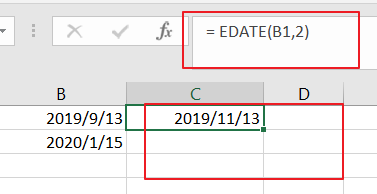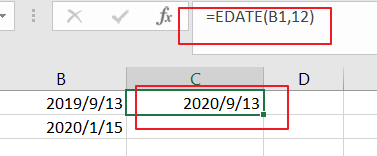# How to Calculate Expiration Dates in Microsoft Excel

This post will guide you how to calculate an expiration in the future in Excel. How do I calculate expiration dates with a formula in Excel.

## Calculate Expiration Dates

Assuming that you have a list of data in range B1:B2, in which contain date values, and you want to calculate the expiration date based on those date. How to accomplish it.

If you want to calculate an expiration in the 30 days, you can use the below formula:

=B1 + 30If you want to calculate an expiration in the two months, you can use the below formula based on the EDATE function, like this:

= EDATE(B1,2)If you want to calculate an expiration in the one year, you can use the following formula, like this:

=EDATE(B1,12)Dates values are serial numbers in Excel, and it based on the year 1900, and the serial number of January 1, 1900 is the number 1. If you want to calculate a date n days in the future, you can add days directly. and If you want to calculate by months, and you can use the EDATE function, and it will return the same date n months in the future.

Sidebar